American Journal of Polymer Science and Technology
Volume 2, Issue 2, November 2016, Pages: 20-27

Investigation of the Factors Effecting on Column Efficiency of Polymer Monolithic Column Using High Performance Liquid Chromatography

Doudou Zhang1, Beijiao Cui1, 2, Xiaoya Jiang1, Ligai Bai1, 2, *, Haiyan Liu1, 2

1College of Pharmaceutical Sciences, Hebei University, Baoding, China

2Key Laboratory of Medicinal Chemistry and Molecular Diagnosis, Ministry of Education, Hebei University, Baoding, China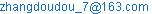(Doudou Zhang)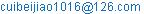(Beijiao Cui)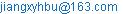(Xiaoya Jiang)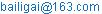(Ligai Bai)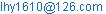(Haiyan Liu)

*Corresponding author

Doudou Zhang, Beijiao Cui, Xiaoya Jiang, Ligai Bai, Haiyan Liu. Investigation of the Factors Effecting on Column Efficiency of Polymer Monolithic Column Using High Performance Liquid Chromatography. American Journal of Polymer Science and Technology. Vol. 2, No. 2, 2016, pp. 20-27. doi: 10.11648/j.ajpst.20160202.11

Received: August 27, 2016; Accepted: September 23, 2016; Published: October 14, 2016

Abstract: In the present work, polymer monolithic columns were prepared via atom transfer radical polymerization technique by using triallyl isocyanurate as monomer, ethylene glycol dimethacrylate as cross linking agent, polyethyleneglycol 200 and 1,4-butylene glycol as binary porogens, N,N-dimethylformamide as solvent, FeCl2 as catalyst, and CCl4 as initiator. Different monolithic columns were obtained by changing the conditions or columns sizes. These columns were used as the stationary phases of high performance liquid chromatography to investigate the factor effecting on the column efficiency. The effects of column diameter (D), pore size (d), porosity (Ö), and linear velocity of the mobile phase (u) on the performance of polymer monolithic columns were studied and the results certified that the plug-like flow in the column was one of the key factors shaping high performance. Furthermore, a structural hydrodynamic model was introduced to investigate the effects of polymer morphology on the performance of liquid chromatography with monolithic columns.

Keywords: Polymer Monolithic Column, Column Efficiency, High Performance Liquid Chromatography, Structural Flow, Atom Transfer Radical Polymerization

1. Introduction

Nowadays, polymer monolithic columns have been developed rapidly and applied widely in high performance liquid chromatography (HPLC) for their special advantages. Though polymer monolithic columns have successfully used in the separation of macro molecular because of their macropores, it is difficult to obtain high column efficiency in the separation of small molecular in conjunction of HPLC.

As we know, a number of processes bring about the broadening of solute bands in column chromatography and a number of theories of band dispersion have been introduced. The most cited example is that of the Knox equation: [1,2]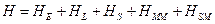(1)

Where HE, HL, HS, HMM, and HSM are as follows: (a) HE, multipath effect; (b) HL, longitudinal diffusion; and (c) HS, HMM, and HSM, resistance to mass transfer. Besides, in order to obtain high performance in liquid chromatography, a packed column, with a diameter of at least 4.6 mm, was usually used for high performance liquid chromatography (HPLC) to avoid the wall effect, which was explained by the "infinite diameter effect" [2,3]. However, we found that, in liquid chromatography, the efficiency of the empty capillary columns by pressure drive is much lower than that of packed ones. The efficiency of packed capillary or monolithic columns is high, in the range of 20 ~ 300 thousand theoretical plates per meter [4-7] and this could not be explained by the Knox equation and the "infinite diameter effect".

There are many factors that affect the performance of liquid chromatography. In this paper, a structural flow hydrodynamic model proposed by Zhou et al , based on the Navier-Stokes equations, is used to investigate the influential factors and to explain how they affect polymer monolithic columns.

In an empty pipe, Newtonian fluid has a zero yield strength and will flow on any slope. But the Newtonian fluid will not flow in porous media, until the initiating pressure is high enough. In liquid chromatography, mobile phase flow in a porous column is plug-like, and that can lead to high performance. The reason is that when initial shear stress is applied to the mobile phase, the flow will not begin immediately . A viscous fluid possesses a yield strength that must be exceeded before the fluid will begin to flow. At the beginning of the flow, only the mobile phase near to the pipe wall can flow, and the fluid at the center can still get together. This is called plug-like flow. There is no gradient in the case of plug-like flow, and the flow rate is the same in every flowing layer. The layers near the pipe wall subsequently begin to flow in order, with the pressure increasing outside. However, fluid at the center of the pipe flows as the same rate as the column at the core (R*). There is no relative flow among the layers of the flowing core, and the fluid flowing with the flowing core is called structural flow. The bigger the flowing core (R*), the more plug-like flow will be formed, and the higher the performance that is obtained.

In the development of the theoretical model, the following conditions are assumed:

a)  The porous structure is uniform

One of the essential factors determining the flow pattern is the geometry of the flow. Therefore, the porous media is a key factor that affects flow geometry and impacts, as a result, the flow pattern in liquid chromatography. Once the flow configuration is achieved, macroscopic turbulence cannot persist in a dense porous media . The uniform dense porous structure is, therefore, the first applicable requirement.

b)  The fluid flowing through the porous media obeys Darcy’s Law

The fluid should be viscid and incompressible, which fit the requirements of Darcy’s Law.

Darcy’s Law is a simple proportional relationship between the instantaneous discharge rate through a porous medium, the viscosity of the fluid, and the pressure drop over a given distance [11-16]. The expression is shown as: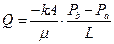(2)

Where the total discharge, Q (units of volume per time, m3/s), is the product of the permeability of the medium k (m2), the cross-sectional area to flow A (units of area, m2), and the pressure drop, all divided by the viscosity μ (Pa·s) and the length L(m) over which the pressure drop is taking place. The negative sign is needed because fluid flows from high to low pressure.

Darcy’s Law is only valid for slow, viscous flow; fortunately, almost all mobile phases in liquid chromatography fall into this category. Typically any flow with a Reynolds number (Re) less than 2 is clearly laminar, and it would be valid to apply Darcy’s Law .

c)  Reynolds number (Re)

According to the previously described conditions that relate to the Reynolds number (Re), when Re<2, the Newtonian fluid flow will be formed through the porous media during structural flow. Moreover, all the following discussions are based on these conditions. The expression of Re is described by: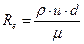(3)

Wherein, ρ is the density of the mobile phase (kg m-3); u is the linear velocity of the mobile phase (m s-1); m is the viscosity coefficient of the mobile phase (Pa s); and d is the pore size (diameter, m). According to (3), in high performance liquid chromatography, when water is used as the mobile phase with a normal velocity (0.001 m s-1), and the pore diameter (d) is 1.0×10-6 m, the Reynolds number (Re) is 1.12×10-3, which is much lower than 2. Therefore, the related conditions in HPLC are all within the reasonable scope.

In the present work, the effects of porosity (Φ), velocity of the mobile phase (u), pore size (d), and column diameter (D) on the column efficiency were studied. A structural hydrodynamic model was introduced in accordance with the performance of polymer monolithic column using as the stationary phase to investigate the effects of polymer morphology on the performance of liquid chromatography with monolithic columns.

2. Experimental

2.1. Material and Instrument

Triallyl isocyanurate (TAIC) and ethylene glycol dimethacrylate (EDMA) were bought from Sigma Chemical Co. (St Louis, MO, USA); Polyethyleneglycol 200 (PEG200), 1,4-butylene glycol, N,N-dimethylformamide (DMF), FeCl2, and CCl4 were purchased from Shanghai Chemical Factory (Shanghai, China); Methanol and acetonitrile were bought from Kermel Chemical Reagent Co. Ltd.(Tianjin, China); Triplex distilled water was used in the experiments; The stainless-steel columns were products of Beijing Xinyu Instrument Co. Ltd. (Beijing, China). Fused-silica capillaries were supplied by the Refine Chromatography Co. Ltd. (Hebei, China).

Agilent 1100 system were applied to HPLC chromatographic studies. Mercury intrusion porosimetry (AutoPore II 9220V 3.04, Micromeritics, USA) was used to assay the porosity, pore distribution, and average pore diameter of the prepared monolithic columns, respectively.

2.2. Preparation and Characterization of the Monolithic Columns

The monoliths were prepared following our previous works [18, 19].

2.2.1. Preparation of the Monolithic Columns

The stainless steel columns sizes were as follows: 50 × 2.1 mm i.d., 50 × 4.0 mm i.d., and 50 × 4.6 mm i.d. (in accordance with columns A – C), respectively. The pre-polymerization solution consisting of TAIC, EDMA, polyethyleneglycol 200, 1,4-butylene glycol, DMF, FeCl2, and CCl4 was poured into the columns to react at 70°C in a water bath for 24 h. We changed the ratios of TAIC/EDMA to prepare monolithic columns (50 × 4.6 mm i.d.) with different pore diameters, which were 3.1, 2.2, and 0.5 μm (in accordance with columns D, C, and E), respectively. The monolithic columns (50 × 4.6 mm i.d.) with different porosities (0.73, 0.65, and 0.56) by changing the amount of porogens, which in accordance with columns C, F, and G in Table 1, respectively. The prepared monolithic columns were all washed online by methanol to remove the porogens. Table 1 listed the component of pre-polymerization solution and pore or column sizes of columns A – G.

2.2.2. Characterization of the Monolithic Columns

Columns A–G were assayed by mercury intrusion porosimetry to demonstrate the pore distribution, average pore diameter, and porosity, respectively. Columns A – G were all tested by HPLC to separate the mixture of benzene, biphenyl, and anthracene to demonstrate their column efficiency, respectively. The chromatographic conditions were as follows: mobile phase: methanol/water; wave length: 254 nm; linear velocity: 0.001 m s-1 (except for there was special caption), temperature: 25°C; sample concentration and injection: 3.0 μL (0.05 mg mL-1).

Table 1. The component of pre-polymerization solution and pore or olumn sizes of columnsa.

 Column TAIC (mL) EDMA (mL) PEG200 (mL) 1,4-butylene glycol (mL) Pore diameter (μm) Column size (internal length × internal diameter, mm) Porosity A 0.5 0.6 0.8 0.4 2.2 50 × 2.1 0.73 B 0.5 0.6 0.8 0.4 2.2 50 × 4.0 0.73 C 0.5 0.6 0.8 0.4 2.2 50 × 4.6 0.73 D 0.7 0.4 0.8 0.4 3.1 50 × 4.6 0.73 E 0.2 0.9 0.8 0.4 0.5 50 × 4.6 0.73 F 0.5 0.6 0.6 0.2 2.2 50 × 4.6 0.65 G 0.5 0.6 0.5 0.1 2.2 50 × 4.6 0.56

a: All the pre-polymerization solutions included the same amount of 0.2 mL DMF, 0.006 g FeCl2, and 0.1mL CCl4, respectively.

3. Results and Discussion

3.1. Theory for the Factor Effecting on Column Efficiency

The hydrodynamic Navier-Stokes equation for the flow of incompressible "Newtonian fluid" in porous media is shown in (4) [8,10]: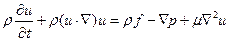(4)

In order to calculate (4), a volume averaged method is used according to the study of Hsu . (5) is obtained and shown below:(5)

This is the generalized expression of the Brinkman-Forchheimer Darcy’s Law that relates to the flow of Newtonian fluids in the porous media, where K is the percolation rate and CF is the inertial coefficient.

In order to solve (5), the force of mass is ignored and Zhou et al  proposed the use of an empirical parabolical equation (6) that is used to express the relationship between the velocity distribution U(R) and the flow core radius R* (dimensionless).(6)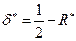(7)

The hydrodynamic equations (8) and (9) are obtained by using (6), (7), and from the solution of (5) .(8)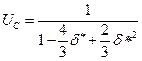(9)

Wherein, δ* is the thickness of boundary layer (dimensionless); K is the percolation rate; D is the character length (diameter of the tube, m); UC is the average flow rate of axial direction (dimensionless); and Φ is the interval porosity.

The thickness of boundary layer (d*) is the important factor that affects fluid mode flow in the tube. The formation of a plug-like flow is due to the smaller thickness of the boundary layer. The plug-like flow of the fluid (mobile phase) is uniform and so there is no spread to the peak, which can lead to high performance in liquid chromatography.

Moreover, the percolation rate K can be calculated by equation (4):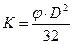(10)

The following equations describe the parameter F:(11)(12)

Wherein, ρ is the density of the mobile phase (kg m-3); u is the linear velocity of the mobile phase (m s-1); m is the viscosity coefficient of the mobile phase (Pa s); and d is the pore size (diameter, m). The equations (3), (8), and (10)-(12) can be grouped into a single equation:

Table 2. The column efficiency of the different columns tested by HPLCb.

 Column A Column B Column C Column D Column E Column F Column G Benzene 2060 2420 7800 4260 9220 7780 7790 Biphenyl 3780 4240 6540 3240 8340 6470 6490 Anthracene 3980 4680 4720 2280 5200 4720 4700

b: The column efficiency were calculated by the formula of theoretical plates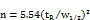(plates m-1).(13)

The flowing core (R*) is directly correlated with the thickness of boundary layer (d*) and the relation was shown in (7). Therefore, the factors that influence the thickness of the boundary layer (d*) also affect on the plug-like flow and then on the column efficiency.

According to (13), the effects of characteristic length (D), pore diameter (d), porosity (Φ), and linear velocity (u) on the column efficiency of polymer monolithic columns were studied, and the results and discussion were as follows.

3.2. The Effects of D, d, Φ, and u on Column Efficiency of Polymer Monolithic Columns

Results obtained from the experimental section 2.2.2 were shown in Table 2, which demonstrated the effects of characteristic length (D), pore diameter (d), and porosity (Φ), respectively. Table 3 showed the column efficiencies obtained from column C using different linear velocities.

3.2.1. Effect of D on the Column Efficiency

D is the character length (diameter of the tube, m). Columns A – C listed in Table 1 were used to investigate the effects of D on the column efficiency. The tube diameters were 2.1, 4.0, and 4.6 mm, respectively. The results shown in Table 2 demonstrated that larger diameter of the tube would lead a higher column efficiency for the polymer monolithic columns, which could be explained by Equation (13) mentioned above.

Figure 1 (A, B) showed the chromatograms and the effect of D on the thickness of boundary layer (d*), respectively. Data shown in Table 2 were in accordance with chromatograms in Figure 1 (A) obtained from columns A-C, which demonstrated that larger diameter would result in higher column efficiency. The reasons were as follows: In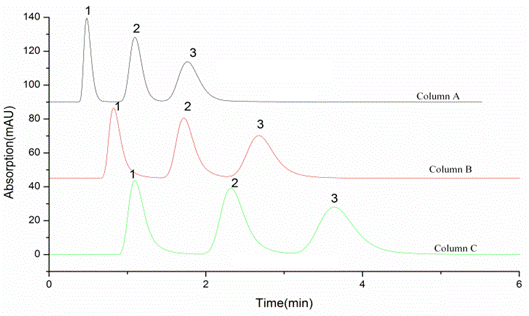(A)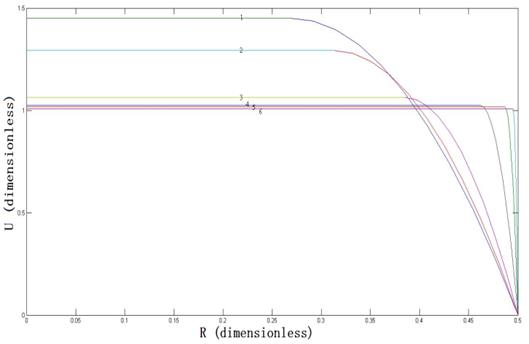(B)

Figure 1. The effects of characteristic length (D) on performance.

(A): Chromatograms from columns A - C

Chromatographic conditions: the column sizes of columns A – C were 50 × 2.1 mm i.d., 50 × 4.0 mm i.d., and 50 × 4.6 mm i.d., respectively; Mobile phase: methanol/water (80/20, v/v); Sample injection: 3.0 μL (0.05 mg mL-1); detection wavelength: 254 nm; Analytes: 1, Benzene; 2, Biphenyl; 3, Anthracene

(B): Model of effects of characteristic length (D) on performance

U is the distribution of axial direction velocity and R is the radius of flow core; The conditions are as follows: d = 2.2 μm; u = 0.001 m s-1; Φ = 0.73; the values of D in Figure 1 (B) (1-6) are: 0.1, 0.15, 0.25, 0.75, 2.1, 4.0, 4.6 mm, in order.

The relationship between D and d* described in Figure 1 (B) showed that the larger D would result in smaller d*, which would then produce a nearer plug-like shape flow. We can, therefore, obtain higher performance by selecting larger characteristic length (D) when the other conditions remain the same. The shape of flow was mostly plug-like when D was 4.6 mm. Therefore, the effects of other parameters on the thickness of boundary layer (d*) were investigated with 4.6 mm being selected as the value of D.

3.2.2. Effect of d on the Column Efficiency

The chromatograms obtained from columns E, C, and D with pore diameters 0.5, 2.2, and 3.1 μm, respectively, were shown in Figure 2 (A). The numbers of theoretical plates for the three columns listed in Table 2 showed that the column with smaller pore diameter had higher column efficiency. Figure 2 (B) showed that when D = 4.6 mm, u = 0.001m s-1, and Φ = 0.73, the decrease in the pore diameter (d) resulted in the decrease of thickness of the boundary layer (d*), and high performance would then be obtained in liquid chromatography following the decrease of the pore diameter (d).

The higher performance of the pores with smaller diameters is usually attributed to a larger specific surface area and the faster mass transfer of the solute. From the present model, one can see that the decrease in the pore diameter leads not only to a faster mass transfer of the solute, but also to more plug-like flow in the column and these, together, increase the performance.

Evidence supporting the model could be found from the experimental results mentioned above. In recent years, considerable efforts have focused on the development of polymer monolithic materials for use in separating small molecules. One major attempt to achieve that was to increase the surface area of porous polymer monolithic columns for the highly efficient separation of small molecules. All the monoliths had high specific surface areas ranging from 370 to 490 m2 g-1, which are obtained by decreasing the pore size and increasing the pore density, and the column diameters ranged from 50 mm to 4.6 mm. The performance was high, in the range of 40~300 thousand theoretical plates per meter [4-7,23-31].

On the previous mechanism , it was important to note that this was only attributed to the higher surface-to- volume ratios of the porous medium, but how the aggravated wall affects the performance of small-diameter columns cannot be clearly explained. However, according to the present theory, the decreased pore size and increased column diameter both result in a more plug-like flow, which contributes to the high performance.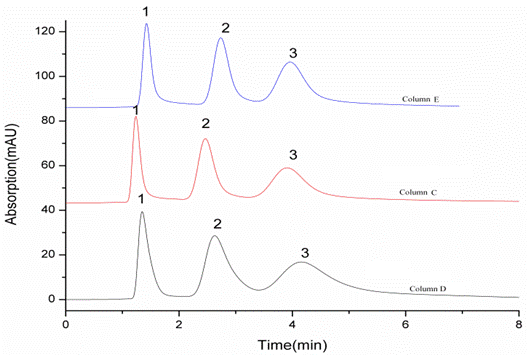(A)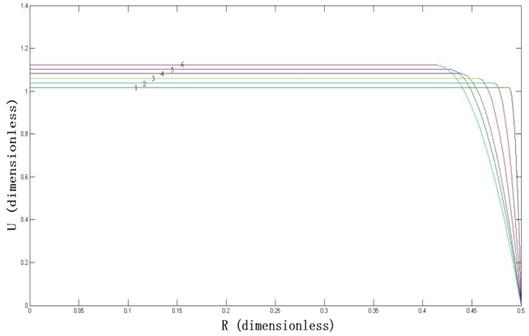(B)

Figure 2. The effects of pore diameter (d) on performance.

(A): Chromatograms from columns C - E

Chromatographic conditions: the column sizes of columns D, C, and E were 50 × 4.6 mm i.d. with pore diameters 3.1, 2.2, 0.5 μm, respectively; Mobile phase: methanol/water (78/22, v/v); Sample injection: 3.0 μL (0.05 mg mL-1); detection wavelength: 254 nm; Analytes: 1, Benzene; 2, Biphenyl; 3, Anthracene

(B): Model of effects of pore diameter (d) on performance

U is the distribution of axial direction velocity and R is the radius of flow core; The conditions are as follows: D = 4.6 mm; u = 0.001 m s-1; Φ = 0.73; the values of d in Figure 2 (B) (1-6) are: 0.2, 1.0, 2.5, 5.0, 7.0, 10.0 μm, in order.

3.2.3. Effect of Φ on the Column Efficiency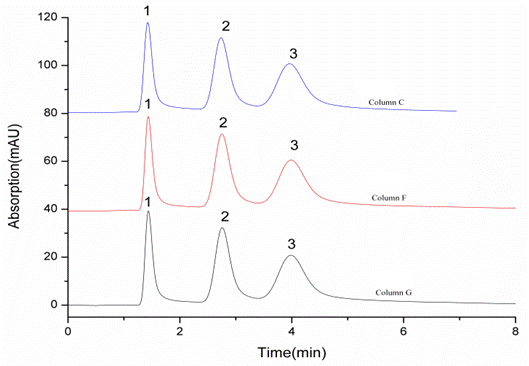(A)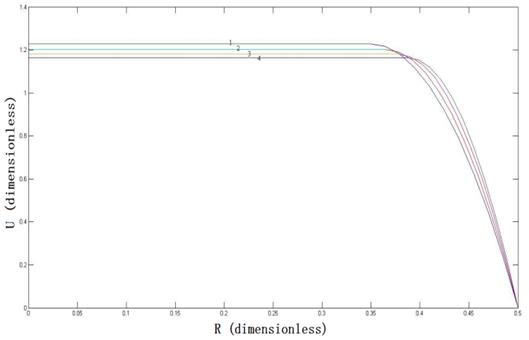(B)

Figure 3. The effects of porosity (Φ) on performance.

(A): Chromatograms from columns C, F, and G

Chromatographic conditions: the column sizes of columns C, F, and G were 50 × 4.6 mm i.d. with porosities 0.73, 0.65, 0.56, respectively; Mobile phase: methanol/water (77/23, v/v); Sample injection: 3.0 μL (0.05 mg mL-1); detection wavelength: 254 nm; Analytes: 1, Benzene; 2, Biphenyl; 3, Anthracene

(B): Model of effects of porosity (Φ) on performance

U is the distribution of axial direction velocity and R is the radius of flow core; The conditions are as follows: D = 4.6 mm; u = 0.001 m s-1; d =2.2 μm; the values of Φ in Figure 3 (B) (1-4) are: 0.60, 0.70, 0.80, 0.90, in order.

Figure 3 (A) obtained from columns C, F, and G, together with the numbers of theoretical plates listed in Table 1 showed that Φ had little effect on the column efficiency. Figure 3 (B) showed the effects of interval porosity (Φ) on the thickness of the boundary layer (d*). The figure showed that when D = 4.6 mm, u = 0.001m s-1, and d = 2.2 μm, the thickness of the boundary layer (d*) did not show any obvious changes following a change in the interval porosity (Φ) from 0.6 to 0.9. This indicated that interval porosity did not remarkably affect column performance.

3.2.4. Effect of u on the Column Efficiency

Figure 4 (A) showed the chromatograms obtained from column C with different linear velocity of the mobile phase. According to the data listed in Table 3, faster linear velocity would lead lower column efficiency because of its larger mass transfer resistance.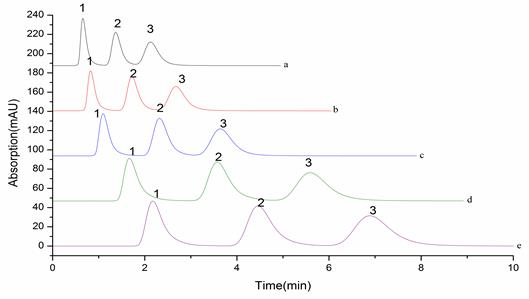(A)(B)

Figure 4. The effects of linear velocity (u) on performance.

(A): Chromatograms from column C

Chromatographic conditions: the column sizes of columns C was 50 × 4.6 mm i.d.; Mobile phase: methanol/water (81/19, v/v); Sample injection: 3.0 μL (0.05 mg mL-1); detection wavelength: 254 nm; linear velocity (a - e): 0.06, 0.08, 0.1, 0.2, 0.5 (×10-2 m s-1), respectively; Analytes: 1, Benzene; 2, Biphenyl; 3, Anthracene

(B): Model of effects of linear velocity (u) on performance

U is the distribution of axial direction velocity and R is the radius of flow core; The conditions are as follows: D = 4.6 mm; d =2.2 μm; Φ = 0.73; the values of u in Figure 4 (B) (1-6) are: 0.01, 0.05, 0. 1, 0. 2, 0. 5, 1.0 (0.01 ×10-2 m s-1), in order.

Figure 4 (B) showed the effect of velocity (u) on the thickness of the boundary layer (d*). The figure shows that when D = 4.6 mm, d = 2.2 μ m, and Φ = 0.73, the increase in the velocity (u) resulted in a decrease in the thickness of the boundary layer (d*). Therefore, the column efficiency would become higher when the velocity was increased in the scope of a laminar flow.

In addition, the viscosity and the density of the mobile phase also both affected on the performance of liquid chromatography. The equation presented in (13) showed that the thickness of boundary layer (d*) was related to the ratio of the viscosity and the mobile phase density. Therefore, the influences that viscosity and mobile phase density exert were not remarkable and were not discussed in detail.

Table 3. The column efficiency of column C tested by HPLC with different linear velocity c, d.

 Velocityd Benzene Biphenyl Anthracene 0.06 7680 6840 5540 0.08 8120 6700 5180 0.1 7800 6540 4720 0.2 7800 6540 4720 0.5 6740 5880 4460

c: The column efficiency was calculated following that of Table 2 (plates m-1);

d: The unit of linear velocity was ×10-2 cm s-1.

4. Conclusions

Together with the data obtained from the polymer monolithic columns, according to the structural flow hydromechanics of incompressible Newtonian fluid in the porous media, the four parameters (D, d, Φ, u) that affected on thickness of boundary layer (d*) affected on column performance. It was certified that the plug-like flow was the key factor that affected on the performance of high performance liquid chromatography with polymer monolithic stationary phase.

Acknowledgment

The authors are grateful for financial support by the National Natural Science Foundation of China (Nos. 21505030, 21175031); the Natural Science Foundation of Hebei Province (No B2015201024); and the National Science Foundation of Hebei University (No. 2014-08).

References

1. J. H. Knox, “Effect of skeleton size on the performance of octadecylsilylated continuous porous silica columns in reversed-phase liquid chromatography,” J. Chromatogr. Sci. vol. 15, pp. 352-364, 1977.
2. G. J. Kennedy, and J. H. J. Knox, “Capacity of sampling and preconcentration columnswith a low number of theoretical plates,” Chromatogr. Sci. vol. 10, pp. 549-556, 1972.
3. J. H. Knox, and J. F. Parcher, “Effect of the column to particle diameter ratio on the dispersion of unsorbed solutes in chromatography,” Anal. Chem. Vol. 41, pp. 1599-1606, 1969.
4. T. Hara, H. Kobayashi, and T. Ikegami, “Performance of monolithic silica capillary columns with increased phase ratios and small-sized domains,” Anal. Chem. vol. 78, pp. 7632-7642, 2006.
5. Y. Ueki, T. Umemura, and J. Li, “Preparation and application of methacrylate-based cation-exchange monolithic columns for capillary ion chromatography,” Anal. Chem. vol. 76, pp. 7007-7012, 2004.
6. M. Wu, R. Wu, and R. Li, “Polyhedral oligomeric silsesquioxane as a cross-linker for preparation of inorganic−organic hybrid monolithic columns,” Anal. Chem. vol. 82, pp. 5447-5454, 2010.
7. B. Gu, Z. Chen, and C. D. “Thulin, efficient polymer monolith for strong cation-exchange capillary liquid chromatography of peptides,” Anal. Chem. vol. 78. pp. 3509-3518, 2006.
8. X. J. Zhou, and Q. B. Guo, “Construction of a fusion bacterial strain and the profile control characteristic of it,” Chin. J. Xian Shiyou Univer. vol. 22, pp. 37-44, 2007.
9. H. Chate, E. Villermaux, and J. M. Chomaz, “In mixing: chaos and turbulence, ”Springer, New York, 1999.
10. B. V. Antohe, and J. L. Lage, “A general two-equation macroscopic turbulence model for incompressible flow in porous media,” J. Heat Mass Transfer vol. 40, pp. 3013-3024, 1997.
11. J. Bear, “Dynamics of fluids in porous media,” Elsevier: New York, 1972.
12. G. Schneebeli, HouilleBlanche, vol. 10, pp. 141-149, 1955.
13. D. E. Wright, and CivilEng. Hydraul. Div. “Nonlinear flow through granular media,” Proc. Am. Soc. vol. 94, pp. 851-872, 1968.
14. J. Comiti, N. E. Sabiri, and A. Montillet, “Experimental characterization of flow regimes in various porous media — III: limit of Darcy's or creeping flow regime for Newtonian and purely viscous non-Newtonian fluids,” Chem. Eng. Sci. vol. 55, pp. 3057-3061, 2000.
15. C. R. Dudgeon, “An experimental study of the flow of water through coarse granular media,” Houille Blanche. vol. 21, pp. 785-801, 1966.
16. G. Chauveteau, and E. L. Darcy, Doctoral Thesis, University of Toulouse, 1965.
17. J. Bear, “Dynamics of Fluids in Porous Media,” Dover Publications, NewYork, 1988, pp. 181
18. H. Zhang, L. Bai, Z. Wei, S. Liu, H. Liu, and H. Yan, “Fabrication of an ionic liquid-based macroporous polymer monolithic column via atom transfer radical polymerization for the separation of small molecules,” Talanta vol. 149, pp. 62-68, 2016.
19. J. Zhong, M. Hao, R. Li, L. Bai, and G. Yang, “Preparation and characterization of poly (triallyl isocyanurate -co- trimethylolpropane triacrylate) monolith and its applications in the separation of small molecules by liquid chromatography,” J. Chromatogr. A vol 1333, pp. 79-86, 2014.
20. L. P. Franca, and S. L. Frey, “Stabilized finite element methods: II. The incompressible Navier-Stokes equations,” Comput. Meth. Appl. Mech. Eng. vol. 99, pp. 209–233, 1992.
21. E. X. Yuan, “Engineering Fluid Mechanics,” Petroleum Industry Press: Beijing, 1986.
22. C. T. Hsu, and P. Cheng, Int. Thermal dispersion in a porous medium, J. Heat Mass Transfer. vol. 33, 1990, pp. 1587-1597.
23. K. Hosoya, N. Hira, and K. Yamamoto, “High-performance polymer-based monolithic capillary column,” Anal. Chem. vol. 78, pp. 5729-5735, 2006.
24. M. Q. Liu, H. Y. Liu, and Y. K. Liu, “Preparation and characterization of temperature-responsive poly (N-isopropylacrylamide-co-N,N′-Methylenebisacrylamide) monolith for HPLC,” J. Chromatogr. A. vol. 1218, pp. 286-292, 2011.
25. Q. Wang, F. Svec, and J. M. J. Fréchet, “Reversed-phase chromatography of small molecules and peptides on a continuous rod of macroporous poly (styrene-co-divinylbenzene),” J. Chromatogr. A. vol. 669, pp. 230-235, 1994.
26. P. Coufal, M. Cihak, and J. Suchankova, “Methacrylate monolithic columns of 320 μm I. D. for capillary liquid chromatography, ” J. Chromatogr. A. vol. 946, pp. 99-106, 2002.
27. J. Lin, J. Lin, X. C. Lin, and Z. H. Xie, “Capillary liquid chromatography using a hydrophilic/cation-exchange monolithic column with a dynamically modified cationic surfactant,” J. Chromatogr. A. vol. 1216, pp. 7728–7731, 2009.
28. J. Urban, F. Svec, and J. M. J. Fréchet, “Hypercrosslinking: New approach to porous polymer monolithic capillary columns with large surface area for the highly efficient separation of small molecules, ” J. Chromatogr. A. vol. 1217, pp. 8212–8221, 2010.
29. Y. Y. Li. H. Dennis Tolley, and M. L. Lee, “Preparation of monoliths from single crosslinking monomers for reversed-phase capillary chromatography of small molecules, ” J. Chromatogr. A. vol. 1218, pp. 1399–1408, 2011.
30. Y. Huo, P. J. Schoenmakers, and W. T. Kok, “Efficiency of methacrylate monolithic columns in reversed-phase liquid chromatographic separations,” J. Chromatogr. A. vol. 1175, pp. 81-88, 2007.
31. K. N. Smirnov, I. A. Dyatchkov, and M. V. Telnov, “Effect of monomer mixture composition on structure and chromatographic properties of poly (divinylbenzene-co-ethylvinylbenzene-co-2-hydroxyethyl methacrylate) monolithic rod columns for separation of small molecules,” J. Chromatogr. A. vol. 1218, pp. 5010-5019, 2011.
32. J. C. Giddings, Dynamics of Chromatography. Part 1. Principles and Theory, Marcel Dekker: NewYork, 1965.

 Contents 1. 2. 2.1. 2.2. 3. 3.1. 3.2. 4.
Article ToolsAbstractPDF(468K)